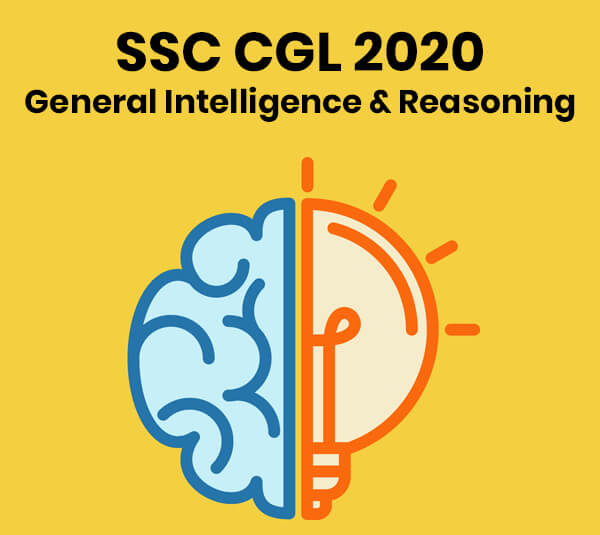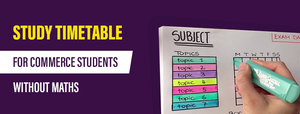General Intelligence & Reasoning - SSC CGL 2020 - Study24x7New to Study24X7 ?

# General Intelligence & Reasoning - SSC CGL 2020

Updated on 15 February 2020SSC 2021 Preparation Strategie
Updated on 15 February 2020The SSC CGL aspirants who are preparing for SSC CGL 2020 must have a look at the following topics from the General Intelligence & Reasoning section of the SSC CGL 2020 syllabus## Classification

The classification topic of the SSC CGL 2020 syllabus deals with the identification of similar types of objects or values on the basis of some common property from a given set of objects or values. We can divide the classification questions in some of the following types:

## Classification Problems on Alphabet

Example: Identify the alphabet which does not belong to the given group in a particular way?

1.  X
2. E
3. I
4. O

Answer: ‘Option a’ is the right answer. As other than ‘X’ all other alphabets are vowels.

## Classification Problems on words

Example: Identify the word which does not belong to the given group in a certain way?

1. Dog
2. Cat
3. Elephant
4. Book

Answer: ‘Option d’ is the right answer. As other than ‘Book’ all other words are the type of animals.

## Classification Problems on Numbers

Example: Identify the number which does not belong to the given set of numbers in a certain way?

1. 12
2. 3
3. 8
4. 26

Answer: ‘Option b’ is the right answer. As other than 3 all the given numbers are even whereas 3 is an odd number.

## Miscellaneous Classification Problems

Example: Identify the option which does not belong to the given set of values in any way?

1. Gold
2. Silver
3. Milk
4. Iron

Answer: ‘Option c’ is the right answer. As other than milk all the options are name of metals.

## Analogy

The analogy questions asked in SSC CGL examination have some kind of given relationship among objects in the question and on the basis of the given relationship the candidate is required to infer another similar type of relationship and information.

Example: In the question, there is a certain relationship between two words. Choose the correct option from the given options which shows the same relationship with the another word associated with it.

Rajasthan: Jaipur:: Maharashtra:?

1. Delhi
2. Mumbai
3. Gwalior

Answer: The right answer is ‘option b’ i.e. Mumbai. Because Jaipur is the capital of Rajasthan and Mumbai is the capital of Maharashtra.

## Coding-Decoding

This topic of non-verbal reasoning section will be asked in SSC CGL 2020 examination to check the ability of the SSC CGL candidate to convert the secret or disguised information into an understandable format or vice versa on the basis of some rule or value work as a secret key.

Here the identification of the secret key is the main problem through which the process of coding and decoding will take place.

Example: If the SEARCH is 346281 and RISK is 2537. Then find the code for RICH?

1. 3561
2. 2581
3. 1674
4. 8321

Answer: The codes of different alphabets on the basis of the given example are:

S=3, E= 4, A=6, R= 2, C= 8, H= 1, I= 5, K= 7

Then the code of RICH in this question will be as 2581.

All The Best To All The SSC CGL 2020 Aspirants !

Write a comment...Trending ArticlesBy CBSE CLASS 8By CBSE CLASS 10By Examination GuideBy K-12-Commerce
Related Posts